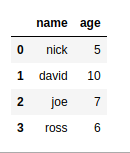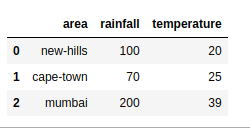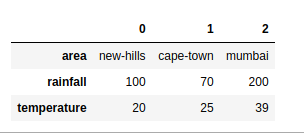Skip to content
Related Articles
How to convert Dictionary to Pandas Dataframe?
• Last Updated : 10 Jul, 2020

Let’s discuss how to convert Python Dictionary to Pandas Dataframe. We can convert a dictionary to a pandas dataframe by using the pd.DataFrame.from_dict() class-method.

Example 1: Passing the key value as a list.

 `import` `pandas as pd`` ` ` ` `data ``=` `{``'name'``: [``'nick'``, ``'david'``, ``'joe'``, ``'ross'``],``        ``'age'``: [``'5'``, ``'10'``, ``'7'``, ``'6'``]} ``new ``=` `pd.DataFrame.from_dict(data)`` ` `new`
Output:Example 2

 `import` `pandas as pd`` ` ` ` `data ``=` `[{``'area'``: ``'new-hills'``, ``'rainfall'``: ``100``, ``'temperature'``: ``20``},``         ``{``'area'``: ``'cape-town'``,  ``'rainfall'``: ``70``, ``'temperature'``: ``25``},``         ``{``'area'``: ``'mumbai'``,  ``'rainfall'``: ``200``,  ``'temperature'``: ``39` `}]`` ` `df ``=` `pd.DataFrame.from_dict(data)`` ` `df`
Output:Example 3: Using the orient parameter to change the orientation of the dataframe from column to index

 `import` `pandas as pd`` ` `data ``=``{``'area'``: [``'new-hills'``, ``'cape-town'``, ``'mumbai'``],``       ``'rainfall'``:[``100``, ``70``, ``200``],``       ``'temperature'``:[``20``, ``25``, ``39``]}`` ` `df ``=` `pd.DataFrame.from_dict(data, orient ``=``'index'``) ``df``print``(df)`
Output:Attention geek! Strengthen your foundations with the Python Programming Foundation Course and learn the basics.

To begin with, your interview preparations Enhance your Data Structures concepts with the Python DS Course. And to begin with your Machine Learning Journey, join the Machine Learning – Basic Level Course

My Personal Notes arrow_drop_up